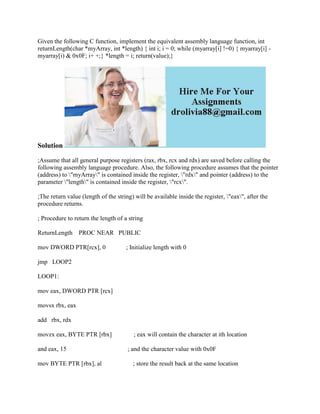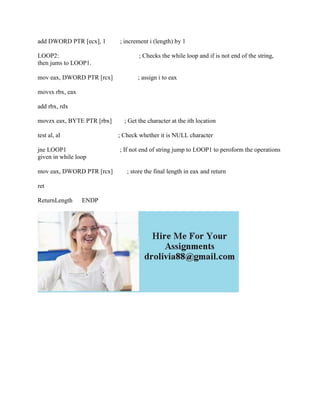Successfully reported this slideshow.

# Given the following C function- implement the equivalent assembly lang.docx×

# Given the following C function- implement the equivalent assembly lang.docx

Given the following C function, implement the equivalent assembly language function, int returnLength(char *myArray, int *length) { int i; i = 0; while (myarray[i] !=0) { myarray[i] - myarray[i) & 0x0F; i+ +;} *length = i; return(value);}
Solution
;Assume that all general purpose registers (rax, rbx, rcx and rdx) are saved before calling the following assembly language procedure. Also, the following procedure assumes that the pointer (address) to \"myArray\" is contained inside the register, \"rdx\" and pointer (address) to the parameter \"length\" is contained inside the register, \"rcx\".
;The return value (length of the string) will be available inside the register, \"eax\", after the procedure returns.
; Procedure to return the length of a string
ReturnLength    PROC NEAR   PUBLIC
mov DWORD PTR[rcx], 0             ; Initialize length with 0
jmp   LOOP2
LOOP1:
mov eax, DWORD PTR [rcx]

movsx rbx, eax
movzx eax, BYTE PTR [rbx]              ; eax will contain the character at ith location
and eax, 15                                       ; and the character value with 0x0F
mov BYTE PTR [rbx], al                    ; store the result back at the same location
add DWORD PTR [ecx], 1         ; increment i (length) by 1

LOOP2:                                                   ; Checks the while loop and if is not end of the string, then jums to LOOP1.
mov eax, DWORD PTR [rcx]                ; assign i to eax

movsx rbx, eax

movzx eax, BYTE PTR [rbx]        ; Get the character at the ith location

test al, al                                     ; Check whether it is NULL character

jne LOOP1                                  ; If not end of string jump to LOOP1 to peroform the operations given in while loop
mov eax, DWORD PTR [rcx]         ; store the final length in eax and return
ret

ReturnLength      ENDP
.

Given the following C function, implement the equivalent assembly language function, int returnLength(char *myArray, int *length) { int i; i = 0; while (myarray[i] !=0) { myarray[i] - myarray[i) & 0x0F; i+ +;} *length = i; return(value);}
Solution
;Assume that all general purpose registers (rax, rbx, rcx and rdx) are saved before calling the following assembly language procedure. Also, the following procedure assumes that the pointer (address) to \"myArray\" is contained inside the register, \"rdx\" and pointer (address) to the parameter \"length\" is contained inside the register, \"rcx\".
;The return value (length of the string) will be available inside the register, \"eax\", after the procedure returns.
; Procedure to return the length of a string
ReturnLength    PROC NEAR   PUBLIC
mov DWORD PTR[rcx], 0             ; Initialize length with 0
jmp   LOOP2
LOOP1:
mov eax, DWORD PTR [rcx]

movsx rbx, eax
movzx eax, BYTE PTR [rbx]              ; eax will contain the character at ith location
and eax, 15                                       ; and the character value with 0x0F
mov BYTE PTR [rbx], al                    ; store the result back at the same location
add DWORD PTR [ecx], 1         ; increment i (length) by 1

LOOP2:                                                   ; Checks the while loop and if is not end of the string, then jums to LOOP1.
mov eax, DWORD PTR [rcx]                ; assign i to eax

movsx rbx, eax

movzx eax, BYTE PTR [rbx]        ; Get the character at the ith location

test al, al                                     ; Check whether it is NULL character

jne LOOP1                                  ; If not end of string jump to LOOP1 to peroform the operations given in while loop
mov eax, DWORD PTR [rcx]         ; store the final length in eax and return
ret

ReturnLength      ENDP
.